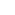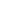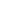# VOCLET 2021 Syllabus, Exam Pattern – Download PDF of West Bengal VOCLET Exam Syllabus

 Details - VOCLET 2021, Application Form, Admit Card, Answer Key, Result Preparation - Syllabus

VOCLET 2021 Syllabus – Get here the West Bengal VOCLET syllabus 2021. You can download the pdf file too to refer to the syllabus, exam pattern at anytime during exam preparation. It is important to study the complete syllabus in order to get a good rank in the Vocational Lateral Entry Test (VOCLET 2021). And to ultimately get admission in college of choice.

## VOCLET 2021 Syllabus

VOCLET Syllabus 2021 PDF: The pdf of VOCLET exam syllabus for 2021 will be available on 25th March.

About the syllabus:

• The West Bengal State Council of Technical Education – WBSCTE (Which is now “West Bengal State Council of Technical & Vocation Education & Skill Development”) prescribes the customized Vocational Lateral Entry Test – VOCLET syllabus every year.
• VOCLET syllabus comprises of Mathematics, Physics, Chemistry, Mechanics and Computer Science subjects. The topics and chapters that are covered in each subject become public through PDF file of the information brochure, that releases on the official site with the declaration of the notification for application.
• The VOCLET 2021 examination will be carried with 100 questions of a total of 100 marks. Out of which 20 questions will be asked from each of the said subjects in the VOCLET syllabus. The exam will be of 2 hours long and asked in Multiple Choice Questions – MCQ type paper. Each correct answer will carry +1 marks. There is a negative marking of 0.25 marks for each incorrect answer.
• Through the VOCLET examination, candidates may get admission direct in 2nd year of polytechnic diploma programmes in the field of Engineering, Pharmacy and Architecture.

## VOCLET 2021 Exam Syllabus: All Important Topics, Subject Wise

The syllabus for 2021 will be available soon. In the meantime you can go through last year’s syllabus given below.

The VOCLET syllabus is containing the Mathematics, Physics, Chemistry, Fundamentals of Mechanics and Computer Applications subjects. Candidates may get the topics-wise syllabus of each subject given below:

### VOCLET 2021 Mathematics Syllabus:

The VOCLET syllabus covers the following Mathematics topics:

Laws of Indices, Complex Numbers, Algebra of Complex Numbers, Cube Roots of unity and its properties, D-Moivre’s Theorem, simple problems.

Sequence and Series, A.P. & G.P. Quadratic Equations, Solutions of Quadratic Equation in the complex number system.

Binomial Theorem, the definition of permutation – combination with formulae, Binomial Theorem for a positive integral index, a general term, the middle term.

Matrix, Determinant, Cramer’s rule [unique solution].

Trigonometric ratios of associated angles: compound angles – multiple and sub-multiple angles, general solution of trigonometric “equation, Inverse circular function.

Co-ordinate system, Distance between two points, section formulae, and formulae for the area of a triangle – problems.

Straight-line – different forms, Angle between two straight lines, condition of parallelism and perpendicular of two straight lines, a distance of a point from a given line.

Standard equation of a circle, simple properties of parabola, ellipse, hyperbola.

Calculus – Function, identity polynomial, rational, modulus and signum functions with their graphs even, odd, periodic and parametric functions.

Differential calculus, limit and continuity, differentiation of parametric, logarithmic and implicit functions, Successive differentiation up to 2nd order problems.

Application of derivatives, Integral calculus: Integration by substitution, by parts, Definite integral – evaluation of definite integral, Application of definite integral.

Differential Equation: solution of a differential equation of 1st order, Solution of a homogeneous differential equation of 1st order and 1st degree.

Vector: addition of vectors, dot product, cross product position vector of a point dividing a line segment in a given ratio.

### VOCLET 2021 Physics Syllabus:

The VOCLET syllabus covers the following Physics topics:

Units of measurement, Dimension of physical quantities.

Motion in a straight line, definitions, Graphical analysis.

Equations of motions, Scalar and vector quantities, Scalar and vector products of vectors.

A frame of reference Newton’s laws, Law of conservation of linear momentum, Friction, Uniform circular motion, vehicle on a level circular road / banked road.

Work, Energy & Power, Centre of mass, Moment / angular momentum, Problems, Moment of inertia and radius of gyration.

Law of gravitation, Gravitational potential, Kepler’s laws of planetary motion, geostationary satellite.

Elastic behaviour, Hooke’s law, Young modulus, bulk modulus, shear modulus of rigidity, Poison’s ratio, elastic energy.

Pascal’s law and its application.

Thermal physics – thermal expansion, ideal gas laws, isothermal and adiabatic processes, anomalous expansion calorimetry, Cp, Cv.

Heat transfer, Newton’s law of cooling, thermal conductivity.

Thermal equilibrium, zeroth law / first law of thermodynamics.

Equation of state of a perfect gas, Kinetic theory of gases.

Periodic motion, Simple harmonic motion [SHM], Simple pendulum, free, damped and forced vibration, resonance.

### VOCLET 2021 Chemistry Syllabus:

The VOCLET syllabus covers the following Chemistry topics:

Chemical Equation, Percentage composition, empirical formula, molecular formula, Problems.

Extranuclear Structure Of Atom: Orbital, subshell, shell. Quantum numbers [n, I, m, s], Pauli Exclusion Principle, Hund’s rule of maximum multiplicity, Auf – bau principle, Ground state electronic configuration of atoms.

Classification of Elements and Periodicity in Properties: present form of the periodic table, S – block and P – block elements, Periodic trend.

Chemical Bonding And Molecular Structure, Hybridization involving s and p orbitals and shapes of some simple molecules, Hydrogen Bond.

Gaseous State Of Matter: numerical problems.

Liquid State of Matter, Thermodynamics  System,  Types of system.

Work, heat, energy, First Law of Thermodynamics – internal energy and enthalpy, Entropy and Gibbs Free Energy, Second Law of Thermodynamics. Significance of the relationG =H – TS.

Equilibrium And Acidimetry – Law of Mass Action, Le Chatelier Principle – simple application, pH and pH scale, Buffer solution, Simple calculation of pH, Acidimetry and Alkalimetry.

Hydrogen, Water: Expression / Estimation of hardness of water, Estimation of hardness of water, Important water quality parameters and their significance.

Hydrogen Peroxide, Preparation and Uses of Sodium carbonate, sodium hydroxide, calcium oxide, bleaching powder, borax, limestone.

Organic Chemistry And Organic Compounds: Estimation of nitrogen [Kjeldahl’s Method], Classification of organic compounds.

IUPAC nomenclature, Alkanes, Alkenes, Alkynes, Arenes, Fuels, Petrochemicals, Lubricants.

Environmental Chemistry: Air Pollution, Water Pollution, Soil Pollution.

### VOCLET 2021 Fundamentals of Mechanics Syllabus:

The VOCLET syllabus covers the following Fundamentals of Mechanics topics:

Concept of Engineering Mechanics: Statics & Dynamics, Scalar quantity, Vector quantity, Addition & subtraction of Vectors.

System of Forces: Definition of Force, System of Co-planner forces, Resultant Force, problems.

Moment & its Applications: Definition& Type, Physical significance, Simple problems.

Equilibrium of Force system: Lami’s theorem, Condition of equilibrium of co-planner & concurrent force / non-concurrent force system, related problems.

Friction: Concept of Friction & its types, Simple problems.

Simple Lifting Machines: Definition of common terms, Study of Simple machines, Simple related problems.

Centre of Gravity: Concept & definition, Formulae for centroids of simple areas, Simple related problems.

Moment of Inertia: Definition, Theorems, Radius of Gyration, related problems.

General Awareness.

### VOCLET 2021 Computer Applications Syllabus:

The VOCLET syllabus covers the following Computer Applications topics:

Introduction to Computer Systems: Definition, Evolution, Generations, Computer Systems, Computer Organization, Input Devices, Output Devices, Computer Memory, Number Systems and Computer Codes.

Computer Software and Programming Languages: Definition and classification of software, DOS, Working with MS-DOS Commands, Algorithm, Flowchart, Concept of High and Low-Level Languages, Windows OS, Word Processing, Spread Sheet.

Internet: Browsing, Use of search engines, E-mail.

Numerical Aptitude.

Note: The official syllabus as prescribed in the last year is given below:

## VOCLET 2021 Exam Pattern

Candidates can read the VOCLET 2021 exam pattern given here:

• Type of Exam: 2nd year Vocational Lateral Entry Test in Polytechnic Diploma programmes.
• Exam Duration: The exam will be organized in a single paper of 2 hours duration.
• Total Questions & Marks: The exam will consist of a total of 100 questions in a total of 100 marks.
• Type of Question: The questions will be asked in MCQ (Multiple Choice Questions) type.
• Mode of Exam: The exam will be organized in offline mode (Paper Based Test).
• Marking Scheme: A +1 mark will be given to each correct answer. However, a 0.25 mark will be deducted for each incorrect answer.
• Syllabus: The syllabus will comprise in Mathematics, Chemistry, Physics, Mechanics and Computer Science subjects.
• Date & Time: The exam will be commenced on 18 July 2021 from 1:30 PM to 3:30 PM.

## VOCLET Preparation Tips

The important preparation tips for the VOCLET 2021 examination are given below:

• Stick to the actual syllabus of the VOCLET examination.
• Make a study time table for your own,
• Collect the best study material before starting your preparation.
• Get the best preparation book from the stationary.
• Enure that your prepared time table must have ample time for your study along with the time for your rejuvenation and rest.
• Don’t forget to revise your studied topics in a week.
• Study in the proper light and airy atmosphere.
• Build you up with physical and mental health.
• Evaluate yourself by solving the sample papers and previous years’ paper.

## FAQs on VOCLET 2021 Syllabus

Ques: What is the syllabus of VOCLET?

Ans: The syllabus prescribed by the WBSCT & VE & Skill Development.

Ques: What subjects to be asked in examinations?

Ans: The examination will comprise in Mathematics, Physics, Chemistry, Fundamentals of Mechanics and Computer Application subjects.

Ques: Where do I get the VOCLET official syllabus?

Ans: The VOCLET topics-wise syllabus will be prescribed by the council through the PDF along with the notification for the application form.

Ques: What books should I study to crack the exam?

Ans: There are many preparation books available on the internet and the book market.

Ques: What subject will cover most of the exam?

Ans:  All subjects will cover the same proportion (20%) of the exam.

Read the above article to get details about VOCLET 2021 syllabus.

Official website: webscte.co.in